# Test: Application Of Integrals (CBSE Level) - 2

## 25 Questions MCQ Test Mathematics (Maths) Class 12 | Test: Application Of Integrals (CBSE Level) - 2

Description
This mock test of Test: Application Of Integrals (CBSE Level) - 2 for JEE helps you for every JEE entrance exam. This contains 25 Multiple Choice Questions for JEE Test: Application Of Integrals (CBSE Level) - 2 (mcq) to study with solutions a complete question bank. The solved questions answers in this Test: Application Of Integrals (CBSE Level) - 2 quiz give you a good mix of easy questions and tough questions. JEE students definitely take this Test: Application Of Integrals (CBSE Level) - 2 exercise for a better result in the exam. You can find other Test: Application Of Integrals (CBSE Level) - 2 extra questions, long questions & short questions for JEE on EduRev as well by searching above.
QUESTION: 1

### The area lying in the first quadrant and bounded by the curve y = x3 , the x – axis and the ordinates at x = - 2 and x = 1 is

Solution:

Required area :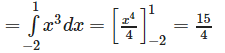QUESTION: 2

### Area bounded by the curves satisfying the conditions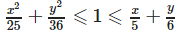is given by

Solution: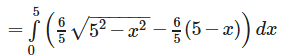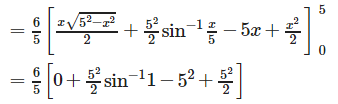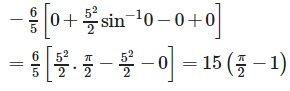QUESTION: 3

### The area of the figure bounded by y = ex, y = e−x and the straight line x = 1 is

Solution: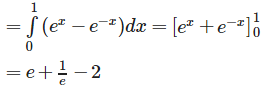QUESTION: 4

The area bounded by the parabolas y = (x+1)2 and y = (x−1)2 and the line y = (1/4) is equal to

Solution:

Required area :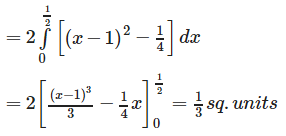QUESTION: 5

The area enclosed between the curve y = loge(x+e) and x = loge 1/y and the x- axes is

Solution:

Required area is :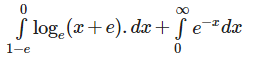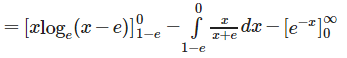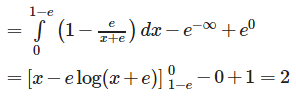QUESTION: 6

The area bounded by the curve y = x3, the x – axis and two ordinates x = 1 and x = 2 is

Solution: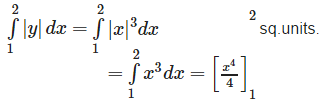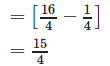QUESTION: 7

The area bounded by the parabola y = x2 + 1 and the straight line x + y = 3 is given by

Solution:

The two curves parabola and the line meet where,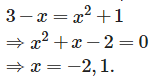Required area ;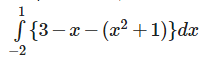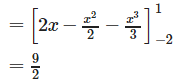QUESTION: 8

The area enclosed between the curves y = √x  , x = 2y+3and the x-axis is

Solution:

The two curves meet where;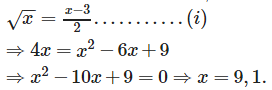Therefore, the two curves meet where x = 9.
Therefore,required Area: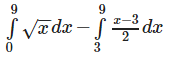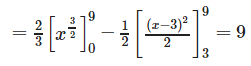QUESTION: 9

The area of the region {(x , y) : x2+y2⩽1⩽x+y} is equal to

Solution:

x2+y2 = 1,x+y = 1
Meets when
x2(1−x)2 = 1
⇒ x2+1+x2−2x = 1
⇒ 2x2−2x= 0 ⇒ 2x(x−1)=0
⇒ x = 0,x = 1.
i.e. points (1 ,0) ,(0 ,1). Therefore , required area is ;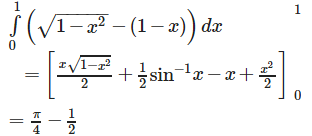QUESTION: 10

The area bounded by y = |sinx| , the x – axis and the line |x| = π is

Solution:

Required area :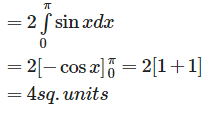QUESTION: 11

If A is the area between the curve y = sin2x , x – axis and the lines x =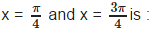Solution:

Required area :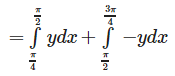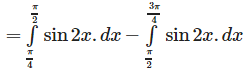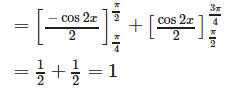QUESTION: 12

The area bounded by the curves y2 = x and y = x2 is

Solution:

The two curves meet in (0 , 0) and (1, 1).The required area lies above the curve y = x2 and below x = y2 and is equal to ;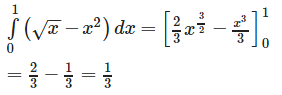QUESTION: 13

The positive value of the parameter a for which the area of the figure bounded by y = sin ax , y = 0 , x = x/a and x = x/3a is 3 is equal to

Solution:

We have :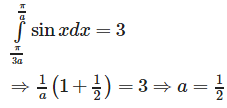QUESTION: 14

The area bounded by the parabolas y = 5x2and y − 9 = 2x2 is

Solution:

Required area :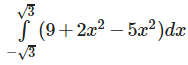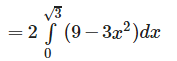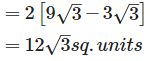QUESTION: 15

The area bounded by the curves y = √x , 2y+3 = x and the x – axis in the first quadrant is

Solution:

Required area :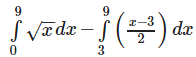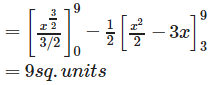QUESTION: 16

The area bounded by y = 2cosx , x = 0 to x = 2π and the axis of x in square units is

Solution:

Required area :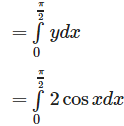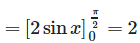Therefore , total area from x = 0 to x = 2π is 4 X 2= 8 sq. units.

QUESTION: 17

The area common to the circle x2+y2 = 16a2 and the parabola y2 = 6ax is

Solution:

Required area :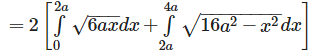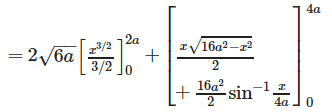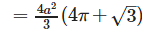QUESTION: 18

The area bounded by the parabola y2 = 4x and the line x + y = 3 is

Solution:

Required area :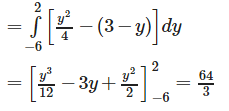QUESTION: 19

The area enclosed by the parabola y2 = 2x and its tangents through the point (-2 , 0) is

Solution: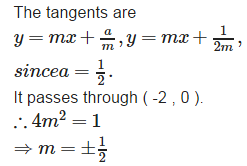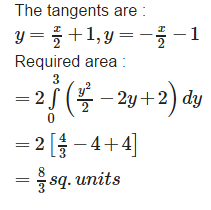QUESTION: 20

The area bounded by the curve y = x log x and  y = 2x−2x2 is

Solution:

Required area :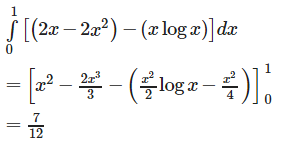QUESTION: 21

The area of the figure bounded by the curve y = logex , the x – axis and the straight line x = e is

Solution:

Required area :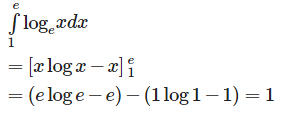QUESTION: 22

The area of the region bounded by the curves y = |x−1| and y = 3 - |x| is

Solution:

Required area :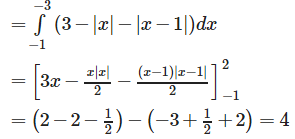QUESTION: 23

The area bounded by the curve y = x2+1and the line x + y = 3 is

Solution:

The two curves parabola and the line meet where,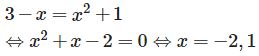Therefore , the required area is :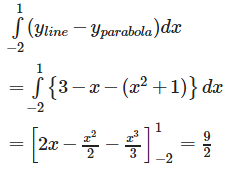QUESTION: 24

The area bounded by the angle bisectors of the lines x2−y2+2y = 1 and x+y = 3 is

Solution:

The angle bisectors of the line given by x2−− y2+2y = 1 are x = 0 , y = 1. Required area = 1/2 .2.2 = 2 sq. units

QUESTION: 25

The area bounded by the curves y= |x−1| and y = 1 is given by

Solution:

The given curves are : (i) y = x – 1 , x > 1 . (ii) y = - (x – 1) , x < 1. (iii) y = 1 these three lines enclose a triangle whose area is : 1/2 .base.height = 1/2 .2 .1 = 1 sq. unit.